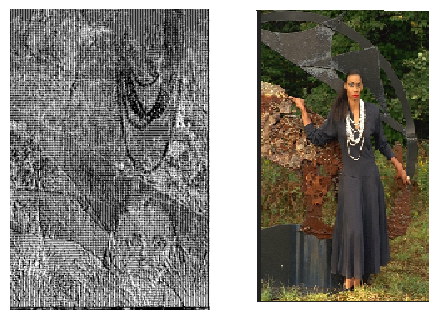# Does convolutional layers convolve the wrong way around

hello
I have been trying to visualize the outputs of a vgg.16 network. But the output seems to be just wrong. As you know the convoltuion doesnt translate the semantic segment of the picture . like for the folwing picture if the head is on the top part of the picture it should be on top of the picture still after the convolution is done . But it doesnt seem to be the case . I used the following code to extract the intermediate layers .

``````class vgg16(torch.nn.Module):
def __init__(self, pretrained=True):
super(vgg16, self).__init__()
vgg_pretrained_features = tv.vgg16(pretrained=pretrained).features
for x in range(30):
def forward(self, x):
output=[]
# print (i)
x=layer(x)
output.append(x)
return output
model=vgg16()
output=model.forward(img)
import matplotlib.pyplot as plt
plt.imshow(output.detach())
``````

here is the original picture and the ouput of the first chanel of the first layer in vgg:As you can see the face has moved all the way down and the neckless is all the way up and the overal structure of the picture is broken

I cannot reproduce the issue and I guess you might be calling `reshape` or `view` on the input image in order to move the channel dimension, which would interleave the image. If that’s the case, use `permute` instead.
For an input ofand this code snippet:

``````class vgg16(torch.nn.Module):
def __init__(self):
super(vgg16, self).__init__()
vgg_pretrained_features = models.vgg16().features
for x in range(30):
def forward(self, x):
output=[]
# print (i)
x=layer(x)
output.append(x)
return output

img = PIL.Image.open('./drums.png')
x = TF.resize(img, (224, 224))
x = TF.to_tensor(x)[None, :3, :, :]

model = vgg16()
output = model(x)

import matplotlib.pyplot as plt
plt.imshow(output.detach())
``````

I get:thank you very much for your time and you are right i did use reshape . Though i dont know why would reshape change the structure of a tensor torch . but thank you anyway .

Assuming you indeed wanted to permute the axes, you could run a simple test via:

``````# setup assuming the image has a shape of [h, w, c]
x = torch.cat((torch.zeros(4, 4, 1), torch.ones(4, 4, 1)), dim=2)

# print channels
print(x[:, :, 0]) # all zeros
print(x[:, :, 1]) # all ones

# wrong approach, which creates an interleaved output
y_wrong = x.view(2, 4, 4)

# print channels
print(y_wrong[0, :, :]) # interleaved
print(y_wrong[1, :, :]) # interleaved

# right approach
y_right = x.permute(2, 0, 1)

# print channels
print(y_right[0, :, :]) # all zeros
print(y_right[1, :, :]) # all ones
``````